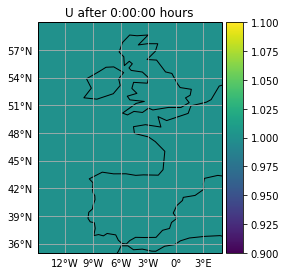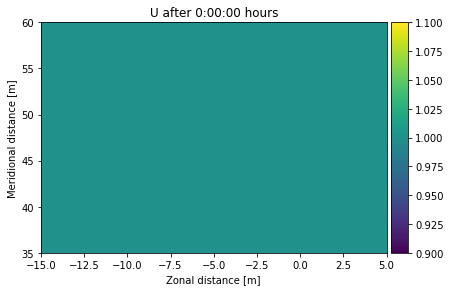## Tutorial on Units and UnitConverters in Parcels¶

In most applications, Parcels works with spherical meshes, where longitude and latitude are given in degrees, while depth is given in meters. But it is also possible to use flat meshes, where longitude and latitude are given in meters (note that the dimensions are then still called longitude and latitude for consistency reasons).

In all cases, velocities are given in m/s. So Parcels seemlessly converts between meters and degrees, under the hood. For transparency, this tutorial explain how this works.

Let's first import the relevant modules, and create dictionaries for the U, V and temp data arrays, with the velocities 1 m/s and the temperature 20C.

In :
%matplotlib inline
from parcels import Field, FieldSet
import numpy as np

In :
xdim, ydim = (10, 20)
data = {'U': np.ones((ydim, xdim), dtype=np.float32),
'V': np.ones((ydim, xdim), dtype=np.float32),
'temp': 20*np.ones((ydim, xdim), dtype=np.float32)}
dims = {'lon': np.linspace(-15, 5, xdim, dtype=np.float32),
'lat': np.linspace(35, 60, ydim, dtype=np.float32)}


We can convert these data and dims to a FieldSet object using FieldSet.from_data. We add the argument mesh='spherical' (this is the default option) to signal that all longitudes and latitudes are in degrees.

Plotting the U field indeed shows a uniform 1m/s eastward flow. The .show() method recognises that this is a spherical mesh and hence plots the northwest European coastlines on top.

In :
fieldset = FieldSet.from_data(data, dims, mesh='spherical')
fieldset.U.show()However, printing the velocites directly shows something perhaps surprising. Here, we use the square-bracket field-interpolation notation to print the field value at (5W, 40N, 0m depth) at time 0.

In :
print((fieldset.U[0, 0, 40, -5], fieldset.V[0, 0, 40, -5], fieldset.temp[0, 0, 40, -5]))

(1.1747725785927632e-05, 8.999280057595393e-06, 20.0)


While the temperature field indeed is 20C, as we defined, these printed velocities are much smaller.

This is because Parcels converts under the hood from m/s to degrees/s. This conversion is done with a UnitConverter object, which is stored in the .units attribute of each Field. Below, we print these

In :
for fld in [fieldset.U, fieldset.V, fieldset.temp]:
print('%s: %s' % (fld.name, fld.units))

U: <parcels.tools.converters.GeographicPolar object at 0x10b550050>
V: <parcels.tools.converters.Geographic object at 0x10b550090>
temp: <parcels.tools.converters.UnitConverter object at 0x311357810>


So the U field has a GeographicPolar UnitConverter object, the V field has a Geographic Unitconverter and the temp field has a UnitConverter object.

Indeed, if we multiply the value of the V field with 1852 * 60 (the number of meters in 1 degree of latitude), we get the expected 1 m/s.

In :
print(fieldset.V[0, 0, 40, -5]* 1852*60)

1.0000000000000002


### UnitConverters for mesh='flat'¶

If longitudes and latitudes are given in meters, rather than degrees, simply add mesh='flat' when creating the FieldSet object.

In :
fieldset_flat = FieldSet.from_data(data, dims, mesh='flat')
fieldset_flat.U.show()
for fld in [fieldset_flat.U, fieldset_flat.V, fieldset_flat.temp]:
print('%s: %f %s' % (fld.name, fld[0, 0, 40, -5], fld.units))U: 1.000000 <parcels.tools.converters.UnitConverter object at 0x1a1e614450>
V: 1.000000 <parcels.tools.converters.UnitConverter object at 0x1a1e6145d0>
temp: 20.000000 <parcels.tools.converters.UnitConverter object at 0x1091cefd0>


Indeed, in this case all Fields have the same default UnitConverter object. Note that the coastlines have also gone in the plot, as .show() recognises that this is a flat mesh.

### UnitConverters for Diffusion fields¶

The units for Brownian diffusion are in $m^2/s$. If (and only if!) the diffusion fields are called kh_zonal and kh_meridional, Parcels will automatically assign the correct Unitconverter objects to these fields.

In :
kh_zonal = 100  # in m^2/s
kh_meridional = 100  # in m^2/s

fieldset.add_field(Field('Kh_zonal', kh_zonal*np.ones((ydim, xdim), dtype=np.float32), grid=fieldset.U.grid))
fieldset.add_field(Field('Kh_meridional', kh_meridional*np.ones((ydim, xdim), dtype=np.float32), grid=fieldset.U.grid))

for fld in [fieldset.Kh_zonal, fieldset.Kh_meridional]:
print('%s: %e %s' % (fld.name, fld[0, 0, 40, -5], fld.units))

Kh_zonal: 1.380091e-08 <parcels.tools.converters.GeographicPolarSquare object at 0x10b5500d0>
Kh_meridional: 8.098704e-09 <parcels.tools.converters.GeographicSquare object at 0x10b550110>


Here, the unitconverters are GeographicPolarSquare and GeographicSquare, respectively.

Indeed, multiplying with $(1852\cdot60)^2$ returns the original value

In :
deg_to_m = 1852*60
print(fieldset.Kh_meridional[0, 0, 40, -5]*deg_to_m**2)

100.0


### Adding a UnitConverter object to a Field¶

So, to summarise, here is a table with all the conversions

Field name Converter object Conversion for mesh='spherical' Conversion for mesh='flat'
'U' GeographicPolar $1852 \cdot 60 \cdot \cos(lat \cdot \frac{\pi}{180})$ 1
'V' Geographic $1852 \cdot 60$ 1
'Kh_zonal' GeographicPolarSquare $(1852 \cdot 60 \cdot \cos(lat \cdot \frac{\pi}{180}))^2$ 1
'Kh_meridional' GeographicSquare $(1852 \cdot 60)^2$ 1
All other fields UnitConverter 1 1

Only four Field names are recognised and assigned an automatic UnitConverter object. This means that things might go very wrong when e.g. a velocity field is not called U or V.

Fortunately, you can always add a UnitConverter later, as explained below:

In :
fieldset.add_field(Field('Ustokes', np.ones((ydim, xdim), dtype=np.float32), grid=fieldset.U.grid))
print(fieldset.Ustokes[0, 0, 40, -5])

1.0


This value for Ustokes of course is not as expected, since the mesh is spherical and hence this would mean 1 degree/s velocity. Assigning the correct GeographicPolar Unitconverter gives

In :
from parcels.tools.converters import GeographicPolar

fieldset.Ustokes.units = GeographicPolar()
print(fieldset.Ustokes[0, 0, 40, -5])
print(fieldset.Ustokes[0, 0, 40, -5]*1852*60*np.cos(40*np.pi/180))

1.1747725785927632e-05
0.9999999999999999


Alternatively, the UnitConverter can be set when the FieldSet or Field is created by using the fieldtype argument (use a dictionary in the case of FieldSet construction.

In :
fieldset.add_field(Field('Ustokes2', np.ones((ydim, xdim), dtype=np.float32), grid=fieldset.U.grid, fieldtype='U'))
print(fieldset.Ustokes2[0, 0, 40, -5])

1.1747725785927632e-05


### Using velocities in units other than m/s¶

Some OGCM store velocity data in units of e.g. cm/s. For these cases, Field objects have a method set_scaling_factor().

If your data is in cm/s and if you want to use the built-in Advection kernels, you will therefore have to use fieldset.U.set_scaling_factor(100) and fieldset.V.set_scaling_factor(100).

In :
fieldset.add_field(Field('Ucm', 0.01*np.ones((ydim, xdim), dtype=np.float32), grid=fieldset.U.grid))
fieldset.Ucm.set_scaling_factor(100)
print(fieldset.Ucm[0, 0, 40, -5])

1.0